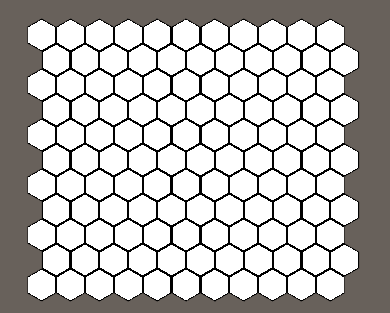HexMapLibrary
Example 0: Hello hex world

The first step of almost every project will be to create the map or board of the game. To do that we first need to import our library into unity (Menubar -> Assets -> Import Package -> Custom Package -> Select the HexMapLibrary.unitypackage file). For now we recommend importing the example folder as they include a hexagon mesh and some other sample prefabs which we use in the following tutorials.

The next step is creating a new script file and reference the needed namespaces of our library:

using System.Collections;
using System.Collections.Generic;

Now we create some variables which we can set in the inspector: the map size and the gameObject we use to represent each tile. [TODO :Explanation of the gameObject and it's material/shader]

[SerializeField] private Vector2Int mapSize = new Vector2Int(11, 11); // the mapSize, can be set in inspector
[SerializeField] private GameObject tilePrefab = null; // the prefab we use for each Tile -> use TilePrefab.prefab [TODO check if that is the correct name]
private HexMap<int> hexMap; // our map. For this example we create a map where an integer represents the data of each tile

Then we can create our map. For this simple example we want each tile to represent an integer and we want the map to be rectangular and do not want it be wrapping around.

void Start ()
{
HexMap = new HexMap<int>(HexMapBuilder.CreateRectangularShapedMap(mapSize), null); //creates a HexMap using one of the pre-defined shapes in the static MapBuilder Class
foreach (var tile in hexMap.Tiles) //loops through all the tiles, assigns them a random value and instantiates and positions a gameObject for each of them.
{
GameObject instance = GameObject.Instantiate(tilePrefab);
instance.transform.position = tile.CartesianPosition;
}
}

Now let's set change the settings of our camera so we can see the whole map and center it:

//put the following at the end of the start method (or in its own method called after map creation)
Camera.main.transform.position = new Vector3(hexMap.MapSizeData.center.x, 4, hexMap.MapSizeData.center.z); // centers the camera and moves it 5 units above the XZ-plane
Camera.main.orthographic = true; //for this example we use an orthographic camera.
Camera.main.transform.rotation = Quaternion.Euler(90, 0, 0); //rotates the camera to it looks at the XZ-plane
Camera.main.orthographicSize = hexMap.MapSizeData.extents.z * 2 * 0.8f; // sets orthographic size of the camera.]
//this does not account for aspect ratio but for our purposes it works good enough.

You should end up with something looking like this:This concludes this example, now let's continue with the next [ADD LINK]